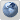# Command that selects Max Value from multiple columns across a row in SQL Server##Command that selects Max Value from multiple columns across a row in SQL Server

I am looking for a function in SLQ Server that essentially acts like COALESCE but instead of selecting the first value that isn't NULL, it selects the maximum value.

I have a process that looks are 4 fields. For each row, I need to pull the maximum value from the 4 fields. Sort of like MAX(Col1, Col2, Col3, Col4), but the MAX function won't do this.

Jeff Smith

Posts : 471
Join date : 2009-02-03

##Re: Command that selects Max Value from multiple columns across a row in SQL Server

Syntax probably not correct, but is this what you are looking for? it would return the largest value from the 4 passed in.

Declare @MX int
SET @MX = -9999
If(@Num1 > @MX) SET @MX = @Num1

If(@Num2 > @MX) SET @MX = @Num2

If(@Num3 > @MX) SET @MX = @Num3

If(@Num4 > @MX) SET @MX = @Num4

Return @MX

TheNJDevil

Posts : 68
Join date : 2011-03-01

##use CASE to test multiple conditions

Very old post but in case someone else stumbles on this, here's a possible way to do this. If none of your values allow for NULL then you can simplify by removing the ISNULL and the ELSE of the case be ELSE Amount4. Beyond that this example works in SQL Server 2008 and above.

Code:
`DECLARE @Purchases TABLE (   ID int IDENTITY(1,1) NOT NULL PRIMARY KEY CLUSTERED,   Amount1 money NOT NULL,   Amount2 money NULL,   Amount3 money NULL,   Amount4 money NULL)INSERT INTO @Purchases (Amount1,Amount2,Amount3,Amount4)VALUES   (10,9,8,NULL),   (1,10,NULL,4),   (1,2,10,3),   (7,8,9,10)SELECT *,Max_Amount =CASE   WHEN (Amount1 >= ISNULL(Amount2,0) AND Amount1 >= ISNULL(Amount3,0) AND Amount1 >= ISNULL(Amount4,0)) THEN Amount1   WHEN (Amount2 >= Amount1 AND Amount2 >= ISNULL(Amount3,0) AND Amount2 >= ISNULL(Amount4,0)) THEN Amount2   WHEN (Amount3 >= Amount1 AND Amount3 >= ISNULL(Amount2,0) AND Amount3 >= ISNULL(Amount4,0)) THEN Amount3   WHEN (Amount4 >= Amount1 AND Amount4 >= ISNULL(Amount2,0) AND Amount4 >= ISNULL(Amount3,0)) THEN Amount4   ELSE NULL --if all 4 values are NULLENDFROM @PurchasesGO`benlotter

Posts : 2
Join date : 2011-08-12
Age : 43
Location : Wisconsin##Re: Command that selects Max Value from multiple columns across a row in SQL Server